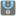• # The Most Numbers. Why isnt this solution accepted?

## Question related to missionThe Most Numbers

```#I get the following errors:
#TypeError: 'float' object is not iterable, checkio, 2
#Fail: checkio(-0.07)

def checkio(*args):
if len(args) > 0: x=max(*args)-min(*args)
else: x=0
return x

#These "asserts" using only for self-checking and not necessary for auto-testing
if __name__ == '__main__':
def almost_equal(checked, correct, significant_digits):
#print(type(checked))
precision = 0.1 ** significant_digits
#print(correct - precision, checked, correct + precision)
return correct - precision < checked < correct + precision

print('Example:')
print(checkio(1, 2, 3))

assert almost_equal(checkio(1, 2, 3), 2, 3), "3-1=2"
assert almost_equal(checkio(5, -5), 10, 3), "5-(-5)=10"
assert almost_equal(checkio(10.2, -2.2, 0, 1.1, 0.5), 12.4, 3), "10.2-(-2.2)=12.4"
assert almost_equal(checkio(), 0, 3), "Empty"
print("Coding complete? Click 'Check' to review your tests and earn cool rewards!")
```